Mathematics is an equally important section for CTET, MPTET, KVS & DSSSB Exams and has even more abundant importance in some other exams conducted by central or state govt. Generally, there are questions asked related to basic concepts of the Simple Interest.

To let you make the most of Mathematics section, we are providing important facts related to the Simple Interest. At least 1-2 questions are asked from Simple Interest topic in most of the teaching exams. We wish you all the best of luck to come over the fear of the Mathematics section.

How to Overcome Exam Fever, Especially When You Fear Maths

## Simple Interest

• Simple interest is nothing but the fixed percentage of the principal (invested/borrowed/ amount of money).
• Principal (P): It is the sum of money deposited/ loaned etc. also known as “Capital”.
• Interest: It is the money paid by the borrower, calculated on the basis of Principal.
• Time (T/n): This is the duration for which money is borrowed.
• Rate of Interest (r/R): It is the rate at which the interest is charged on principal.
• Amount (A) = Principal + Interest

Mathematics Study Notes For All Teaching Exams

Some Basic Formulae :

Simple Interest (SI):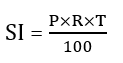P = Principal,

r = rate of interest (in %)

t = time period (yearly, half yearly etc.)Some Useful Short-cut Methods:

1. If a certain sum in T years at R % per annum amounts to Rs. A, then the sum will be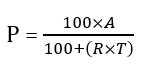1. If a certain sum is invested in n types of investments in such a manner that equal amount is obtained on each investment where interest rates are R₁R₂ R₃ …..Rn respectively and time periods are T₁ T₂ T₃….. Tn respectively, then the ratio in which the amounts are invested is :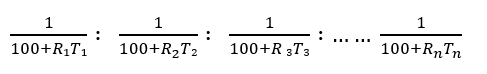How To Score 25+ Marks In Mathematics For CTET Exam?

1. If a certain sum of money becomes n times itself in T years at simple interest, then the rate of interest per annum is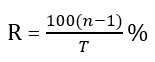1. If a certain sum of money becomes n times itself in T years at a simple interest, then the time T in which it will become m times itself is given by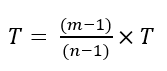Mathematics Quiz For CTET Exam : Attempt Daily Quizzes

1. Effect of change of P, R and T on simple interest is given by the following formulae: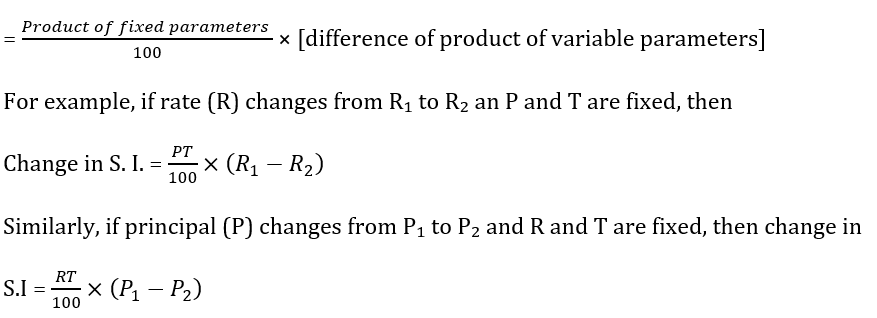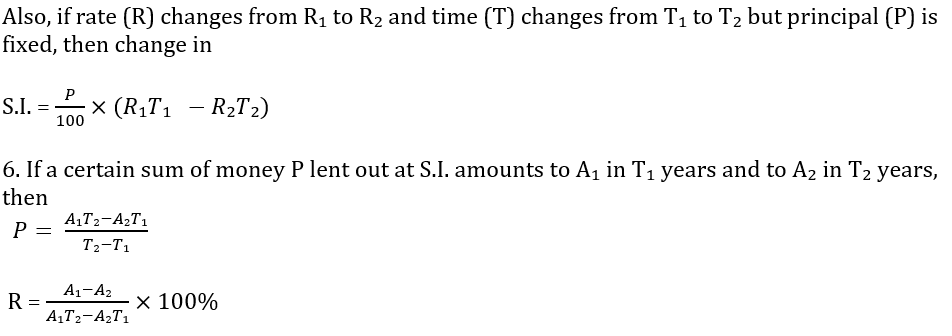×

Thank You, Your details have been submitted we will get back to you.Join India's largest learning destination

What You Will get ?

•Job Alerts
•Daily Quizzes
•Subject-Wise Quizzes
•Current Affairs
•Previous year question papers
•Doubt Solving session

ORJoin India's largest learning destination

What You Will get ?

•Job Alerts
•Daily Quizzes
•Subject-Wise Quizzes
•Current Affairs
•Previous year question papers
•Doubt Solving session

ORJoin India's largest learning destination

What You Will get ?

•Job Alerts
•Daily Quizzes
•Subject-Wise Quizzes
•Current Affairs
•Previous year question papers
•Doubt Solving session

Enter the email address associated with your account, and we'll email you an OTP to verify it's you.Join India's largest learning destination

What You Will get ?

•Job Alerts
•Daily Quizzes
•Subject-Wise Quizzes
•Current Affairs
•Previous year question papers
•Doubt Solving session

Enter OTP

Please enter the OTP sent to
/6

Did not recive OTP?

Resend in 60sJoin India's largest learning destination

What You Will get ?

•Job Alerts
•Daily Quizzes
•Subject-Wise Quizzes
•Current Affairs
•Previous year question papers
•Doubt Solving sessionJoin India's largest learning destination

What You Will get ?

•Job Alerts
•Daily Quizzes
•Subject-Wise Quizzes
•Current Affairs
•Previous year question papers
•Doubt Solving session

Almost there

+91Join India's largest learning destination

What You Will get ?

•Job Alerts
•Daily Quizzes
•Subject-Wise Quizzes
•Current Affairs
•Previous year question papers
•Doubt Solving session

Enter OTP

Please enter the OTP sent to Edit Number

Did not recive OTP?

Resend 60

By skipping this step you will not recieve any free content avalaible on adda247, also you will miss onto notification and job alerts

Are you sure you want to skip this step?

By skipping this step you will not recieve any free content avalaible on adda247, also you will miss onto notification and job alerts

Are you sure you want to skip this step?